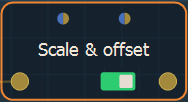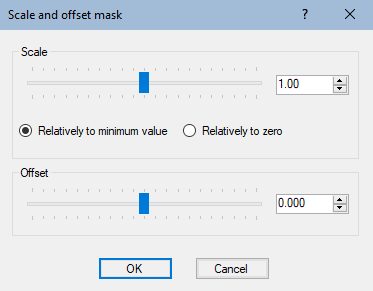Adding a Scale & offset node

This node sets the scale and offset of the mask.

To add a Scale & offset node, right click in the Graph Editor and select Create Node  > Mask Transformation  > Scale & offset.Double click on the node to open its parameters:Editing the scale and offset

• Use the Scale slider to change the scale of the mask size.
• Set to what the scale is relative:
• Relative to minimum height: Minimum value of the mask heights does not change. If the mask heights are between -20 and 80 (range 100), and with a factor of 2, the heights are between -20 and 180 (range 200).
• Relatively to zero: Zero heights do not change. If the mask heights are between -20 and 80 (range 100), and with a factor of 2, the heights are between -40 and 160 (range 200).
• Offset: Sets the positive or negative offset to apply.

Parameters

Parameter Use
Scale Scale factor to be applied.
Relatively to minimum height Minimum value of the mask heights does not change. If the mask heights are between -20 and 80 (range 100), and with a factor of 2, the heights are between -20 and 180 (range 200).
Relatively to zero Zero heights do not change. If the mask heights are between -20 and 80 (range 100), and with a factor of 2, the heights are between -40 and 160 (range 200).
Offset The offset (positive or negative) to apply.